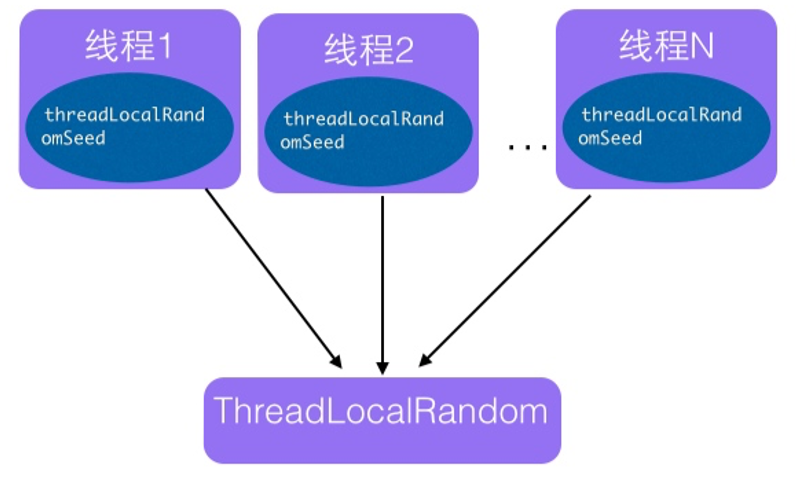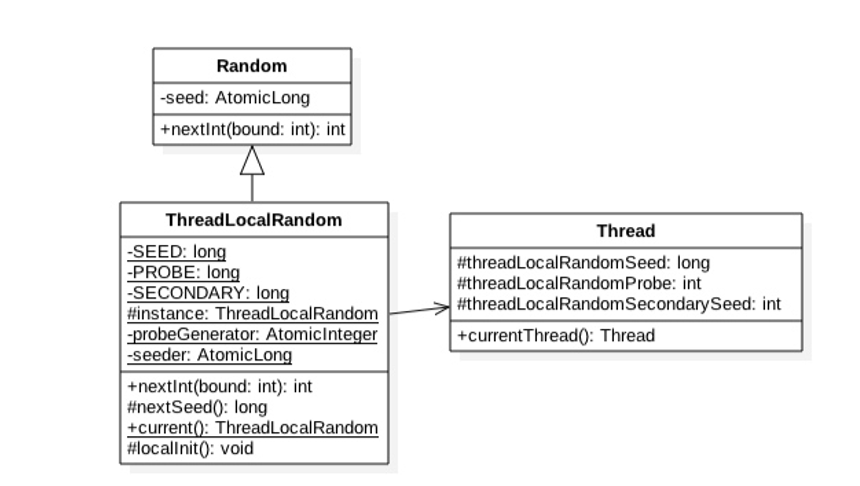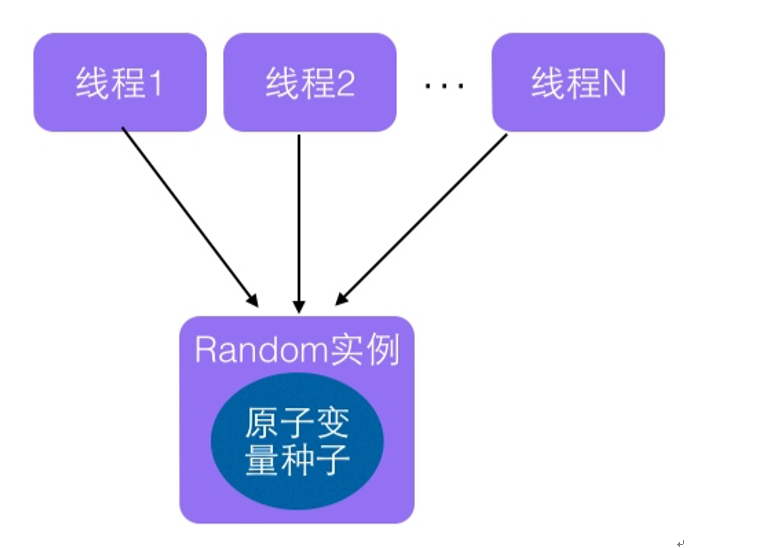在 JDK7 之前包括现在，java.util.Random 应该是使用比较广泛的随机数生成工具类，另外 java.lang.Math 中的随机数生成也是使用的 java.util.Random 的实例。下面先看看 java.util.Random 的使用例子如下：

/**
* Created by cong on 2018/6/4.
*/
public class RandomTest {

public static void main(String[] args) {

//(1)创建一个默认种子的随机数生成器
Random random = new Random();
//(2)输出10个在0-5（包含0，不包含5）之间的随机数
for (int i = 0; i < 10; ++i) {
System.out.println(random.nextInt(5));
}

}
}public int nextInt(int var1) {　　　　//（3）参数校验
if(var1 <= 0) {
throw new IllegalArgumentException("bound must be positive");
} else {　　　　　　　//（4）根据老的种子生成心的种子
int var2 = this.next(31);　　　　　　　//（5）以下根据新的种子计算随机数
int var3 = var1 - 1;
if((var1 & var3) == 0) {
var2 = (int)((long)var1 * (long)var2 >> 31);
} else {
for(int var4 = var2; var4 - (var2 = var4 % var1) + var3 < 0; var4 = this.next(31)) {
;
}
}

return var2;
}
}

1.首先需要根据老的种子生成新的种子。

2.然后根据新的种子来计算新的随机数。

Random 函数使用一个原子变量达到了这个效果，在创建 Random 对象时候初始化的种子就保存到了种子原子变量里面，下面看下 next() 的源码：

 protected int next(int bits) {
long oldseed, nextseed;
AtomicLong seed = this.seed;
do {
//(6)
oldseed = seed.get();
//(7)
//(8)
} while (!seed.compareAndSet(oldseed, nextseed));
//(9)
return (int)(nextseed >>> (48 - bits));
}private static final sun.misc.Unsafe UNSAFE;
private static final long SEED;
private static final long PROBE;
private static final long SECONDARY;
static {
try {
//获取unsafe实例
UNSAFE = sun.misc.Unsafe.getUnsafe();
SEED = UNSAFE.objectFieldOffset
PROBE = UNSAFE.objectFieldOffset
SECONDARY = UNSAFE.objectFieldOffset
} catch (Exception e) {
throw new Error(e);
}
}

　　static final ThreadLocalRandom instance = new ThreadLocalRandom();
//(12)
//(13)
localInit();
//(14)
return instance;
}
static final void localInit() {
int probe = (p == 0) ? 1 : p; // skip 0
UNSAFE.putLong(t, SEED, seed);
UNSAFE.putInt(t, PROBE, probe);
}

int nextInt(int bound) 方法：计算当前线程的下一个随机数。源码如下图所示：

 public int nextInt(int bound) {
//(15)参数校验
if (bound <= 0)
//(16) 根据当前线程中种子计算新种子
int r = mix32(nextSeed());
//(17)根据新种子和bound计算随机数
int m = bound - 1;
if ((bound & m) == 0) // power of two
r &= m;
else { // reject over-represented candidates
for (int u = r >>> 1;
u + m - (r = u % bound) < 0;
u = mix32(nextSeed()) >>> 1)
;
}
return r;
}

　　final long nextSeed() {
return r;
}

public class RandomTest {

public static void main(String[] args) {
//(10)获取一个随机数生成器

//(11)输出10个在0-5（包含0，不包含5）之间的随机数
for (int i = 0; i < 10; ++i) {
System.out.println(random.nextInt(5));
}
}
}

运行结果如下：posted @ 2018-06-04 15:49  妮蔻  阅读(511)  评论(0编辑  收藏